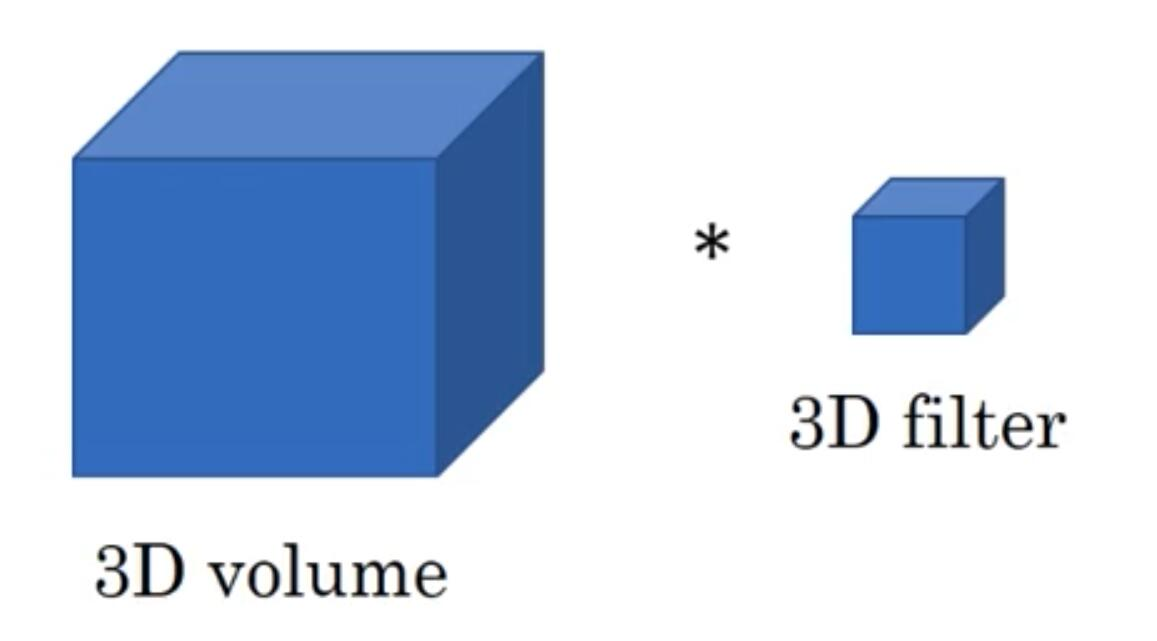﻿ 基于Keras中Conv1D和Conv2D的区别说明_python_脚本之家
python# 基于Keras中Conv1D和Conv2D的区别说明

```x = tf.nn.convolution(
input=x,
filter=kernel,
dilation_rate=(dilation_rate,),
strides=(strides,),
data_format=tf_data_format)```

```self.kernel_size = conv_utils.normalize_tuple(kernel_size, rank, 'kernel_size')
……#中间代码省略
input_dim = input_shape[channel_axis]
kernel_shape = self.kernel_size + (input_dim, self.filters)```

```def normalize_tuple(value, n, name):
"""Transforms a single int or iterable of ints into an int tuple.
# Arguments
value: The value to validate and convert. Could an int, or any iterable
of ints.
n: The size of the tuple to be returned.
name: The name of the argument being validated, e.g. "strides" or
"kernel_size". This is only used to format error messages.
# Returns
A tuple of n integers.
# Raises
ValueError: If something else than an int/long or iterable thereof was
passed.
"""
if isinstance(value, int):
return (value,) * n
else:
try:
value_tuple = tuple(value)
except TypeError:
raise ValueError('The `' + name + '` argument must be a tuple of ' +
str(n) + ' integers. Received: ' + str(value))
if len(value_tuple) != n:
raise ValueError('The `' + name + '` argument must be a tuple of ' +
str(n) + ' integers. Received: ' + str(value))
for single_value in value_tuple:
try:
int(single_value)
except ValueError:
raise ValueError('The `' + name + '` argument must be a tuple of ' +
str(n) + ' integers. Received: ' + str(value) + ' '
'including element ' + str(single_value) + ' of type' +
' ' + str(type(single_value)))
return value_tuple
```

input_dim = input_shape[channel_axis] kernel_shape = self.kernel_size + (input_dim, self.filters)

（3,300,64）

（3,3,1,64）

（3,300,1,64），也即这个时候的Conv1D的大小reshape一下得到，二者等价。

1. 二维卷积2. 一维卷积3. 三维卷积